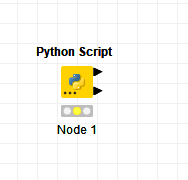# python script - pandas dataframe index problem

Trying to read a special file format with a python lib that I’ve found. Seems to work so far, as the generated csv-file for debugging purpose seems correct.

Screenshot:Here is my script:

``````import numpy as np
import pandas as pd
df = pd.DataFrame()

from nptdms import TdmsFile

for group in tdms_file.groups():
group_name = group.name
for channel in group.channels():
df[channel] = channel[:].tolist()

#df.to_csv("C:/temp/df.csv")
output_table_1 = df
``````

Yet the node does not work:Any ideas?

did the to_csv work?
Have you tried printing it to see whether the table is returned? Is in an option to change the data type of the column?
br

This package seems to produce quite complicated column names. I think they cannot be easily converted to knime column names.

1 Like

Hi @Thoru ,

I have some ideas on what you might be able to do, but I’m struggling to test the theory without a tdms file. The only one I found on the internet was 45MB and by the time I’d loaded it into a dataframe in KNIME it was causing my installation to stutter considerably!

My idea is this. Add a second output port to your KNIME Python Script node.Rename the columns for the dataframe going to the first node so that they are just column1, column2… etc

Send a list of the column names derived from the the tdms file to the second output port.

That way, you would have a readable data set but with standardised column names, and then a second dataset containing the column names from the file. After that, maybe you can manipulate the list of column names into a form that works with KNIME, and rename the columns in the first data set as appropriate.

Your script would be something like this:

``````import numpy as np
import pandas as pd
df = pd.DataFrame()

from nptdms import TdmsFile

for group in tdms_file.groups():
print(group)
group_name = group.name
for channel in group.channels():
df[channel] = channel[:].tolist()

# get list of column names from dataframe
column_names=[col for col in df]

# rename the column names to be output in table 1 as
# Column1, Column2, Column3,... ColumnN

df.columns = ["Column" + str(i) for i,x in enumerate(df.columns)]
output_table_1 = df

# Output captured list of columns to output_table_2
output_table_2=pd.DataFrame(column_names)
``````

I haven’t been able to test the above script. If you are able to upload a small sample tdms file in a sample workflow, further help may be available.

5 Likes

By the way, @Thoru , are you sure the following line

`df[channel] = channel[:].tolist()`

shouldn’t say

`df[group_name] = channel[:].tolist()`

I don’t know anything about the format of tdms files, or your data but that looks odd to me (and was the reason why my KNIME was running out of memory!!), and might explain the problem you’ve been having. Just a thought!

If that turns out to be the issue, you can ignore the rest of my script above as it may not be required!1 Like

Thanks a lot! The strange names were the issue.

A column name is for example `<TdmsChannel with path /'abc-11'/'U_MOT'>`
I only need the last part `U_MOT` as column name.

Here is my working script:

``````import numpy as np
import pandas as pd
df = pd.DataFrame()

from nptdms import TdmsFile

for group in tdms_file.groups():
group_name = group.name
for channel in group.channels():
df[channel] = channel[:].tolist()

#df.to_csv("C:/temp/df.csv")
#column_names=[col for col in df]
#for c in column_names:
#    print(str(type(c))+", "+str(c)+", "+str(c).split("/")[-1][1:-2])

df.columns=[str(col).split("/")[-1][1:-2] for col in df]
output_table_1 = df
``````
5 Likes

This topic was automatically closed 7 days after the last reply. New replies are no longer allowed.